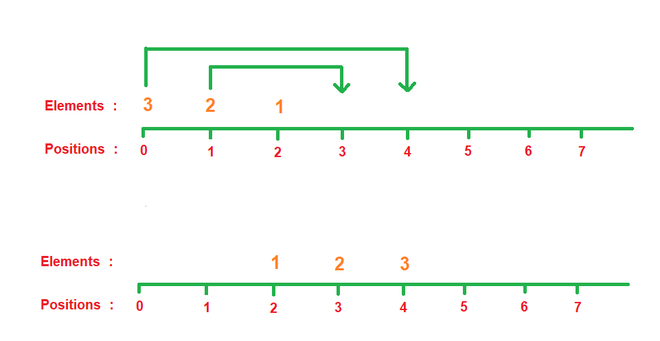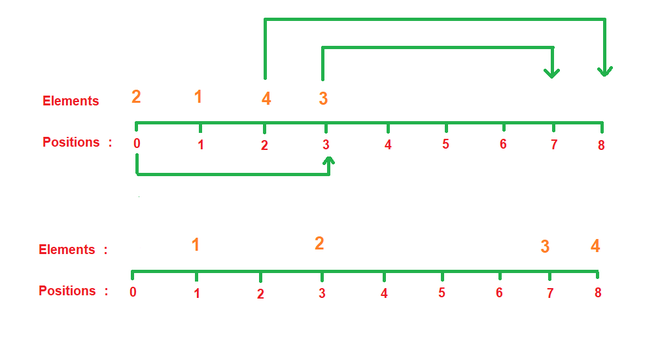Open in App
Not now

# Sort the elements by minimum number of operations

• Last Updated : 20 Mar, 2023

Given two positive integer arrays X[] and Y[] of size N, Where all elements of X[] are distinct. Considering all the elements of X[] are lying side by side initially on a line, the task is to find the minimum number of operations required such that elements in X[] becomes in increasing order where in one operation you can increase each element of X[] by their respective values index in Y[] (first element of X[] element can be incremented by the first element of Y[] or position of the second element of X[] can be incremented by the second element of Y[] or so on….).

Examples:

Input: N = 3, X[] = {3, 2, 1}, Y[] = {1, 1, 1}
Output: 6
Explanation: According to the problem statement:

• X = 3, It’s position can be incremented by Y = 1.
• X = 2, It’s position can be incremented by Y = 1.
• X = 1, It’s position can be incremented by Y = 1.
• Operations:
• In two operations, X = 2, incremented its position by Y = 1 in each operation from position 1 to 2 and then 2 to 3 by using operations one by one.
• In four operations, X = 3, incremented its position by Y = 1 in each operation from position 0 to 1, 1 to 2, 2 to 3 and then 3 to 4 in last operation. Each operation is performed one by one.
• Now, It can be verified that all the elements of X[] are in sorted order of their values, for this case Minimum number of operations are=2 + 4 = 6, Which is minimum possible.Teset case 1

Input: N = 4, X[] = {2, 1, 4, 3}, Y[] = {4, 1, 2, 4}
Output: 5
Explanation: According to the problem statement:

•  X = 2, It’s position can be incremented by Y = 4.
•  X = 1, It’s position can be incremented by Y = 1.
•  X = 4, It’s position can be incremented by Y = 2.
•  X = 3, It’s position can be incremented by Y = 4.
• Operations:
• In one operation, X = 3, incremented its position by Y = 4, in each operation from position 3 to 7.
• In one operation, X = 2, incremented its position by Y = 4 in each operation from position 0 to 3.
• In three operations, X = 4, incremented its position by Y = 2 in each operation from position 2 to 4, 4 to 6 and then 6 to 8 in each operation one by one.
• Therefore, Total minimum number of operations for this case are= 1 + 1 + 3 = 5. It can be verified that all the elements of X[] are in sorted order of their values after performing above operations.Test case 2

Approach: Implement the idea below to solve the problem

The problem is observation based and can be solve by using Greedy Technique. The basic idea of the problem is that, we have to choose optimal element to increment it’s position in ach operation.

Steps were taken to solve the problem:

• Create two arrays positions[] and temp_length[] of length N for storing positions and temporary lengths of elements of X[].
• Create integer variable operations and initialize it equal to 0.
• Initialize positions[] with positions[X[i] – 1] = i,   by using a loop from i=0 to less than N.
• Initialize temp_length[] with temp_lengthX[i] – 1] = Y[i],  by using a loop from i=0 to less than N.
• Iterate from i = 1 to less than N using loops and follow the below-mentioned steps under the scope of the loop:
• While(position[i] ≤ position[i-1]), till then position[i] += temp_length[i] and operations++.
• Return operations.

Below is the code to implement the approach:

## C++

 `// C++ code to implement the approach` `#include ` `using` `namespace` `std;`   `// Function for returning minimum` `// number of operations` `int` `min_operations(``int` `N, ``int` `X[], ``int` `Y[])` `{`   `    ``// Array to store positions` `    ``// of elements` `    ``int` `position[N];`   `    ``// Array to store temp_length` `    ``int` `temp_length[N];`   `    ``// Variable to hold minimum number` `    ``// of operations` `    ``int` `operations = 0;`   `    ``// Loop for initializing positions` `    ``for` `(``int` `i = 0; i < N; i++) {` `        ``position[X[i] - 1] = i;` `    ``}`   `    ``// Loop for initializing` `    ``// temp_length` `    ``for` `(``int` `i = 0; i < N; i++) {` `        ``temp_length[X[i] - 1] = Y[i];` `    ``}`   `    ``// Loop for calculating number` `    ``// of operations` `    ``for` `(``int` `i = 1; i < N; i++) {`   `        ``while` `(position[i] <= position[i - 1]) {` `            ``position[i] += temp_length[i];` `            ``operations++;` `        ``}` `    ``}`   `    ``// Returning number of operations` `    ``return` `operations;` `}`   `int` `main() {`   `      ``// Input value of N` `    ``int` `N = 4;`   `    ``// Input array X[]` `    ``int` `X[] = { 2, 1, 4, 3 };`   `    ``// Input array Y[]` `    ``int` `Y[] = { 4, 1, 2, 4 };`   `    ``// Function call` `     ``cout << min_operations(N, X, Y) << endl;` `}`

## Java

 `// Java code to implement the approach`   `import` `java.io.*;` `import` `java.lang.*;` `import` `java.util.*;`   `class` `GFG {`   `    ``// Driver Function` `    ``public` `static` `void` `main(String[] args)` `        ``throws` `java.lang.Exception` `    ``{`   `        ``// Input value of N` `        ``int` `N = ``4``;`   `        ``// Input array X[]` `        ``int` `X[] = { ``2``, ``1``, ``4``, ``3` `};`   `        ``// Input array Y[]` `        ``int` `Y[] = { ``4``, ``1``, ``2``, ``4` `};`   `        ``// Function call` `        ``System.out.println(min_operations(N, X, Y));` `    ``}`   `    ``// Function for returning minimum` `    ``// number of operations` `    ``static` `int` `min_operations(``int` `N, ``int``[] X, ``int``[] Y)` `    ``{`   `        ``// Array to store positions` `        ``// of elements` `        ``int``[] position = ``new` `int``[N];`   `        ``// Array to store temp_length` `        ``int``[] temp_length = ``new` `int``[N];`   `        ``// Variable to hold minimum number` `        ``// of operations` `        ``int` `operations = ``0``;`   `        ``// Loop for initializing positions` `        ``for` `(``int` `i = ``0``; i < N; i++) {` `            ``position[X[i] - ``1``] = i;` `        ``}`   `        ``// Loop for initializing` `        ``// temp_length` `        ``for` `(``int` `i = ``0``; i < N; i++) {` `            ``temp_length[X[i] - ``1``] = Y[i];` `        ``}`   `        ``// Loop for calculating number` `        ``// of operations` `        ``for` `(``int` `i = ``1``; i < N; i++) {`   `            ``while` `(position[i] <= position[i - ``1``]) {` `                ``position[i] += temp_length[i];` `                ``operations++;` `            ``}` `        ``}`   `        ``// Returning number of operations` `        ``return` `operations;` `    ``}` `}`

## Python3

 `#Python3 code to implement the approach`   `# Function for returning minimum` `# number of operations` `def` `min_operations(N, X, Y):`   `    ``# Array to store positions` `    ``# of elements` `    ``position ``=` `[``0``] ``*` `N`   `    ``#Array to store temp_length` `    ``temp_length ``=` `[``0``] ``*` `N`   `    ``# Variable to hold minimum number` `    ``# of operations` `    ``operations ``=` `0`   `    ``#Loop for initializing positions` `    ``for` `i ``in` `range``(``0``, N):` `        ``position[X[i] ``-` `1``] ``=` `i`   `    ``# Loop for initializing` `    ``# temp_length` `    ``for` `i ``in` `range``(``0``, N):` `        ``temp_length[X[i] ``-` `1``] ``=` `Y[i]`   `    ``# Loop for calculating number` `    ``# of operations` `    ``for` `i ``in` `range``(``1``, N):`   `        ``while` `position[i] <``=` `position[i ``-` `1``]:` `            ``position[i] ``+``=` `temp_length[i]` `            ``operations ``+``=` `1`   `    ``# Returning number of operations` `    ``return` `operations`   `if` `__name__ ``=``=` `"__main__"``:`   `    ``# Input value of N` `    ``N ``=` `4`   `    ``#Input array X[]` `    ``X ``=` `[ ``2``, ``1``, ``4``, ``3` `]`   `    ``# Input array Y[]` `    ``Y ``=` `[ ``4``, ``1``, ``2``, ``4` `]`   `    ``# Function call` `    ``print``(min_operations(N, X, Y))`

## C#

 `// C# code to implement the approach` `using` `System;` `public` `class` `GFG {`   `  ``static` `public` `void` `Main()` `  ``{`   `    ``// Code` `    ``// Input value of N` `    ``int` `N = 4;`   `    ``// Input array X[]` `    ``int``[] X = { 2, 1, 4, 3 };`   `    ``// Input array Y[]` `    ``int``[] Y = { 4, 1, 2, 4 };`   `    ``// Function call` `    ``Console.WriteLine(min_operations(N, X, Y));` `  ``}`   `  ``// Function for returning minimum` `  ``// number of operations` `  ``static` `int` `min_operations(``int` `N, ``int``[] X, ``int``[] Y)` `  ``{`   `    ``// Array to store positions` `    ``// of elements` `    ``int``[] position = ``new` `int``[N];`   `    ``// Array to store temp_length` `    ``int``[] temp_length = ``new` `int``[N];`   `    ``// Variable to hold minimum number` `    ``// of operations` `    ``int` `operations = 0;`   `    ``// Loop for initializing positions` `    ``for` `(``int` `i = 0; i < N; i++) {` `      ``position[X[i] - 1] = i;` `    ``}`   `    ``// Loop for initializing` `    ``// temp_length` `    ``for` `(``int` `i = 0; i < N; i++) {` `      ``temp_length[X[i] - 1] = Y[i];` `    ``}`   `    ``// Loop for calculating number` `    ``// of operations` `    ``for` `(``int` `i = 1; i < N; i++) {`   `      ``while` `(position[i] <= position[i - 1]) {` `        ``position[i] += temp_length[i];` `        ``operations++;` `      ``}` `    ``}`   `    ``// Returning number of operations` `    ``return` `operations;` `  ``}` `}`   `// This code is contributed by karthik.`

## Javascript

 `// Javascript code to implement the approach`   `// Function for returning minimum` `// number of operations` `function` `min_operations( N,  X,  Y)` `{`   `    ``// Array to store positions` `    ``// of elements` `    ``let position=``new` `Array(N);`   `    ``// Array to store temp_length` `    ``let temp_length=``new` `Array(N);`   `    ``// Variable to hold minimum number` `    ``// of operations` `    ``let operations = 0;`   `    ``// Loop for initializing positions` `    ``for` `(let i = 0; i < N; i++) {` `        ``position[X[i] - 1] = i;` `    ``}`   `    ``// Loop for initializing` `    ``// temp_length` `    ``for` `(let i = 0; i < N; i++) {` `        ``temp_length[X[i] - 1] = Y[i];` `    ``}`   `    ``// Loop for calculating number` `    ``// of operations` `    ``for` `(let i = 1; i < N; i++) {`   `        ``while` `(position[i] <= position[i - 1]) {` `            ``position[i] += temp_length[i];` `            ``operations++;` `        ``}` `    ``}`   `    ``// Returning number of operations` `    ``return` `operations;` `}`   `// Input value of N` `let N = 4;`   `// Input array X[]` `let X = [ 2, 1, 4, 3 ];`   `// Input array Y[]` `let Y = [ 4, 1, 2, 4 ];`   `// Function call` `console.log(min_operations(N, X, Y));`   `// This code is contributed by ratiagrawal.`

Output

`5`

Time Complexity: O(N)
Auxiliary Space: O(N)

My Personal Notes arrow_drop_up
Related Articles